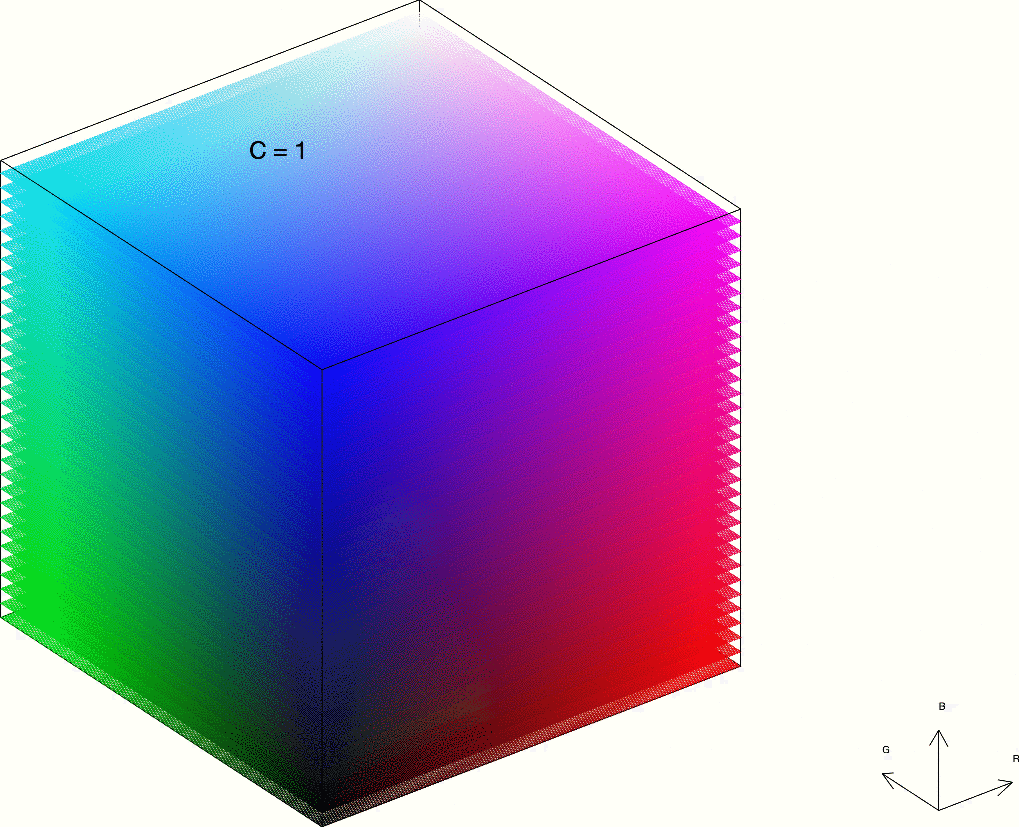# Color Contrast

On Both Black and WhitePhoto by Paweł Czerwiński on Unsplash
This post is an adaptation/extension of the excellent post by Ben Szabo on dev.to.
If you want to know more about web accessibility I recommend you take a look at this excellent guide.
Do you want to know even more about color schemes and how to choose an aesthetic color palette in 2021? Check out this awesome blog post from Evinex.

I am considering the possibility of allowing the visitors of my website to choose whether they want a light or dark background. With a dark background (almost black) the text would become light (almost white), the opposite of what it is currently, but the accent color, this blue , would no longer have enough contrast on black.

## What is contrast and how is it defined?

The Web Content Accessibility Guidelines (WCAG) define the contrast ratio, $C$, as:

$$C = \frac{L1 + 0.05}{L2 + 0.05},$$

where $L1$ y $L2$ are the relative luminances of the lighter and darker colors, respectively. The relative luminance is defined as:

The relative brightness of any point in a colorspace, normalized to 0 for darkest black and 1 for lightest white.

In the case of the sRGB color space, the default used throughout the Web, there are some simple expressions to calculate this relative luminance, which depends on the coordinates of the color in question.

Black has a relative luminance equal to 0, while that of white is equal to 1, so the maximum possible contrast, $C_\text{max}$, is 1:

$$C_\text{max} = \frac{1+0.05}{0+0.05} = 21$$

It is easy to see that the minimum contrast is equal to 1, since it is given when both colors have the same relative luminosity ($L1 = L2$).

The WCAG guidelines say that the minimum contrast ratio between a given text and its background should be at least 4.5:1.

Colorable is an excellent tool with which we can check the contrast of color combinations.

## So, which colors look good on both black and white?

Given the relative luminance of a color, $L$, we can calculate its contrast on black, $C_\text{black}$, with the expression2:

$$C_\text{black} = \frac{L + 0.05}{0 + 0.05} = \frac{L+0.05}{0.05}$$

Contrast on white, $C_\text{white}$, is calculated with the expression3:

$$C_\text{white} = \frac{1 + 0.05}{L + 0.05} = \frac{1.05}{L+0.05}$$

If we want to choose a color that looks good on both black and white, we must impose that both contrasts, $C_\text{black}$ and $C_\text{white}$, are at least equal to 4.5. That gives us these two inequalities:

\begin{align*} C_\text{black} &= \frac{L+0.05}{0.05} \geq 4.5 \tag{1} \\
C_\text{white} &= \frac{1.05}{L+0.05} \geq 4.5 \tag{2} \end{align*}

From the first one (black) we get:

$$L \geq 4.5\cdot 0.05-0.05 = 0.175$$

whereas from the second one (white):

$$L \leq \frac{1.05}{4.5}-0.05 = 0.18\overline{3},$$

So that $0.175\leq L\leq 0.18\overline{3}$.

Ben Szabo has created this Pen which iterates through the RGB color space, with increments of 17 per channel4, listing 76 colors whose contrast on both black and white is at least 4.5.

See the Pen Colors With 4.5:1 Contrast on Black and White by Ben Szabo (@finnhvman) on CodePen.

## What is the maximum contrast we can achieve on both black and white?

In order to have enough contrast on both black and white, the relative luminance of the color has to be between two values5, as you can see with the inequalities (1) and (2). The theoretical maximum contrast of a color on both black and white, $C_\text{b&w}^\text{max}$, can be obtained by imposing that those two values of relative luminance be equal:

$$\frac{1.05}{C_\text{b&w}^\text{max}}-\cancel{0.05} = C_\text{b&w}^\text{max}\cdot 0.05-\cancel{0.05},$$

from where we get $C_\text{b&w}^\text{max} = \sqrt{21} \approx 4.58$, which corresponds to this fuchsia color:

• #cf0dcc
rgb(207,13,204)

A good combination of primary colors (red, green and blue) would be6:

• #e62101
rgb(230,33,1)
• #038901
rgb(3,137,1)
• #2f72de
rgb(47,114,222)

## But, what if we show all the available colors?

In his Pen, Ben Szabo iterates through the RGB color space, with increments of 17 per channel, warning us that, if we were to iterate through all possible colors (increments of 1), we would get ~300k colors. Obviously listing so many colors does not seem very practical, but visualizing them can be very interesting.

The following figure shows how many colors are available depending on the desired contrast:

We can see how for $C=1$ (the minimum possible contrast), all the colors in the RGB space are available, namely:

$$256\cdot 256\cdot 256 = 16\thinspace 777\thinspace 216$$

colors. As we increase the contrast, the number of colors decreases, plummeting from $C=4.5$, until we have only one color available in the limit $C_\text{b&w}^\text{max} = \sqrt{21}$, the fuchsia #cf0dcc.

The following animation shows all the colors available in the RGB space (a 256-sided cube) depending on the desired contrast (on both black and white):RGB colors available depending on the desired contrast, ranging from $C=1$ ($256\cdot 256\cdot 256 = 16\thinspace 777\thinspace 216$ available colors) to the limit $C_\text{b&w}^\text{max}=\sqrt{21}$ in which we only have the fuchsia color #cf0dcc.

1. As white is lighter than black, its luminosity, 1, goes in the numerator, while that of black, 0, goes in the denominator. ↩︎

2. The color in question will be the lightest color ($L$ in the numerator), since black is the darkest color. ↩︎

3. As white is the lightest color, $L$ goes in the denominator. ↩︎

4. In the RGB color model, values for each channel (red, green and blue) vary from 0 to 255. Increments of 17 allow for iterating through the colors that can be described with 3-digit hexa notation. ↩︎

5. In other words, the color does not have to be either very light (poor contrast on white) or very dark (poor contrast on black). ↩︎

6. All three with a contrast close to the theoretical maximum of $\sqrt{21}$ on both black and white. ↩︎##### Rodrigo Alcaraz de la Osa
###### PhD in Physics and Physics and Chemistry Teacher

I teach Physics and Chemistry at IES Ría San Martín in Cantabria (Spain).